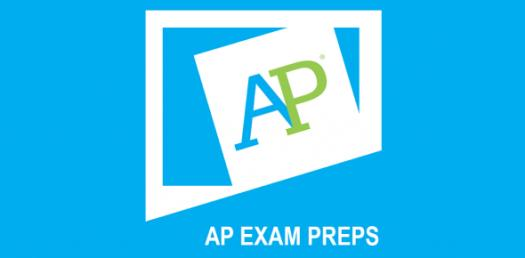# Statistics Hardest Questions: Advanced Placement Exam! Trivia Quiz

Approved & Edited by ProProfs Editorial Team
At ProProfs Quizzes, our dedicated in-house team of experts takes pride in their work. With a sharp eye for detail, they meticulously review each quiz. This ensures that every quiz, taken by over 100 million users, meets our standards of accuracy, clarity, and engagement.
| Written by Gian Petersen
G
Gian Petersen
Community Contributor
Total Contribution - 14 | Total attempts - 8,165
Questions: 5 | Attempts: 513SettingsThe trivia quiz below is Statistics Hardest Questions from the Advanced Placement Exam! It is designed to give students an idea of how the exams are set up and at the same time measure what they can remember from their classwork. If you need a quiz to do all this for you, be sure to take this comprehensive and challenging quiz.

• 1.

### A university is proposing a new procedure for professors to gain tenure.  To gauge sentiment about the proposal, the university intends to randomly sample five professors, five assistant professors, and five associate professors.  This is an example of what type of sampling design?

• A.

Simple random sample

• B.

Stratified random sample

• C.

Systematic random sample

• D.

Cluster sample

• E.

Convenience sample

B. Stratified random sample
Explanation
The university's proposal to randomly sample professors, assistant professors, and associate professors is an example of a stratified random sample. In this sampling design, the population is divided into distinct groups or strata (in this case, the different professor ranks), and a random sample is taken from each stratum. This ensures that each group is represented in the sample proportionally to its size in the population, allowing for more accurate conclusions about the sentiment towards the tenure proposal among different professor ranks.

Rate this question:

• 2.

### Twenty types of 12 oz. cans of soda were tested for calories and sugar (mg).  The sodas averaged 156.85 calories with a standard deviation of 22.64, and the sugar level averaged 401.15 mg with a standard deviation of 102.43 mg.  The correlation was given as r = 0.887.  The equation of the LSRL predicting sugar level from calories is:

• A.

Predicted y = -228.30 + 4.01x

• B.

Predicted y = 22.64 - 156.85x

• C.

Predicted y = 102.43 - 401.15x

• D.

Predicted y = 4.01 - 228.33x

• E.

Predicted y = 78.19 + 0.196x

A. Predicted y = -228.30 + 4.01x
Explanation
The equation of the least squares regression line (LSRL) is used to predict the value of the dependent variable (sugar level) based on the independent variable (calories). In this case, the LSRL equation is predicted y = -228.30 + 4.01x. This means that for every unit increase in calories, the predicted sugar level increases by 4.01 units. The intercept of -228.30 represents the predicted sugar level when the calories are zero.

Rate this question:

• 3.

### A random variable has a standard deviation of 1.3.  A new variable is created by transforming the values of the random variable using the following rule:  Multiply each value by 2 and then add 5.  What is the value of the standard deviation for this transformed variable?

• A.

1.3

• B.

2.6

• C.

6.3

• D.

7.6

• E.

8.5

B. 2.6
Explanation
When a random variable is transformed by multiplying each value by a constant and then adding another constant, the standard deviation of the transformed variable is equal to the product of the original standard deviation and the absolute value of the multiplying constant. In this case, the multiplying constant is 2, so the standard deviation of the transformed variable is 1.3 * 2 = 2.6.

Rate this question:

• 4.

### An airline has an on-time probability of 82.4%.  What is the probability that, if you travel on this airline, no more than three of your next ten flights will not be on time? (Assume the flights are independent.) Select an answer.

• A.

A

• B.

B

• C.

C

• D.

D

• E.

E

E. E
Explanation
The probability that no more than three out of ten flights will not be on time can be calculated using the binomial probability formula. The formula is P(X ≤ k) = Σ (n choose i) * p^i * (1-p)^(n-i), where n is the number of trials, i is the number of successes, p is the probability of success, and (n choose i) is the binomial coefficient. In this case, n = 10, i can be 0, 1, 2, or 3, p = 0.824, and (n choose i) can be calculated using the binomial coefficient formula. By plugging in these values and summing up the probabilities for i = 0, 1, 2, and 3, we can find the probability that no more than three flights will not be on time.

Rate this question:

• 5.

### A random sample of 25 birth-weights (in ounces) is taken, yielding the following summary statistics. What can be said about the number of outliers for this data set?

• A.

0

• B.

At least 1

• C.

No more than 1

• D.

At least 2

• E.

No more than 2Back to top